Subsections

# 6.2 BinarySearchTree: An Unbalanced Binary Search Tree

A BinarySearchTree is a special kind of binary tree in which each node,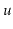, also stores a data value,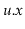, from some total order. The data values in a binary search tree obey the binary search tree property: For a node,, every data value stored in the subtree rooted at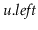is less thanand every data value stored in the subtree rooted at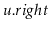is greater than. An example of a BinarySearchTree is shown in Figure 6.5.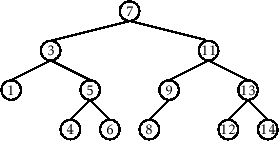## 6.2.1 Searching

The binary search tree property is extremely useful because it allows us to quickly locate a value,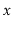, in a binary search tree. To do this we start searching forat the root,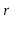. When examining a node,, there are three cases:

1. If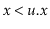, then the search proceeds to;
2. If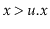, then the search proceeds to;
3. If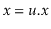, then we have found the nodecontaining.
The search terminates when Case 3 occurs or when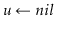. In the former case, we found. In the latter case, we conclude thatis not in the binary search tree.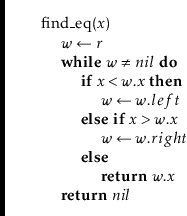Two examples of searches in a binary search tree are shown in Figure 6.6. As the second example shows, even if we don't findin the tree, we still gain some valuable information. If we look at the last node,, at which Case 1 occurred, we see thatis the smallest value in the tree that is greater than. Similarly, the last node at which Case 2 occurred contains the largest value in the tree that is less than. Therefore, by keeping track of the last node,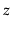, at which Case 1 occurs, a BinarySearchTree can implement the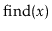operation that returns the smallest value stored in the tree that is greater than or equal to: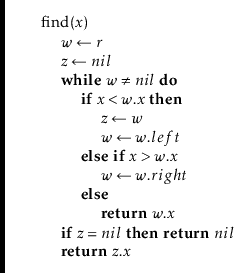Figure 6.6: An example of (a) a successful search (for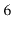) and (b) an unsuccessful search (for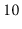) in a binary search tree.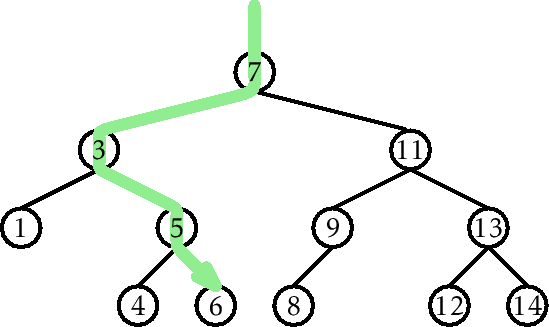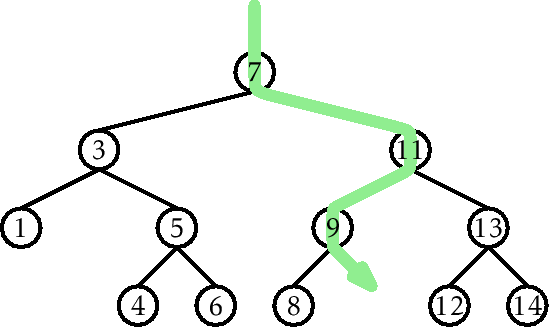(a) (b)

To add a new value,, to a BinarySearchTree, we first search for. If we find it, then there is no need to insert it. Otherwise, we storeat a leaf child of the last node,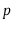, encountered during the search for. Whether the new node is the left or right child ofdepends on the result of comparingand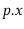.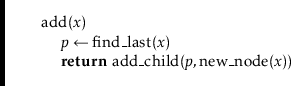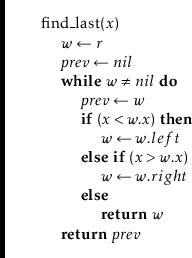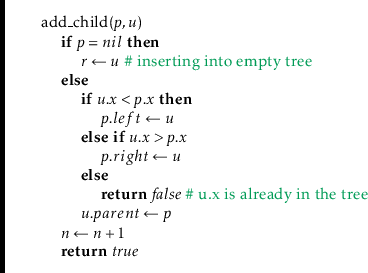An example is shown in Figure 6.7. The most time-consuming part of this process is the initial search for, which takes an amount of time proportional to the height of the newly added node. In the worst case, this is equal to the height of the BinarySearchTree.

Figure 6.7: Inserting the value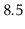into a binary search tree.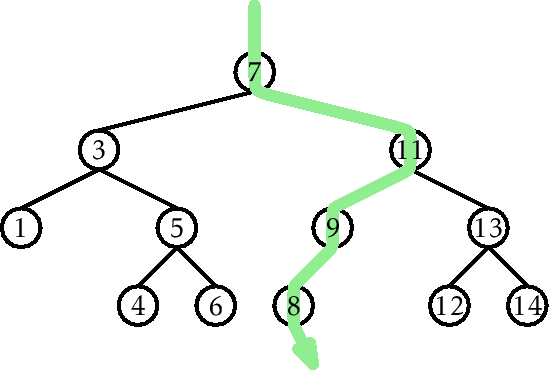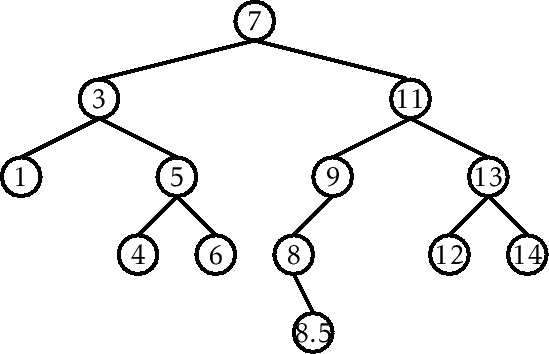## 6.2.3 Removal

Deleting a value stored in a node,, of a BinarySearchTree is a little more difficult. Ifis a leaf, then we can just detachfrom its parent. Even better: Ifhas only one child, then we can splicefrom the tree by having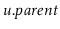adopt's child (see Figure 6.8):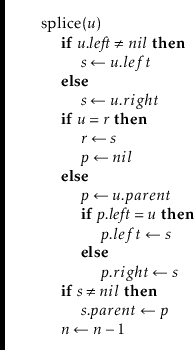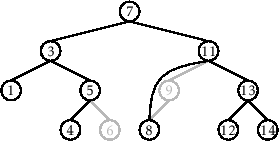Things get tricky, though, whenhas two children. In this case, the simplest thing to do is to find a node,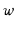, that has less than two children and such thatcan replace. To maintain the binary search tree property, the value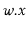should be close to the value of. For example, choosingsuch thatis the smallest value greater thanwill work. Finding the nodeis easy; it is the smallest value in the subtree rooted at. This node can be easily removed because it has no left child (see Figure 6.9).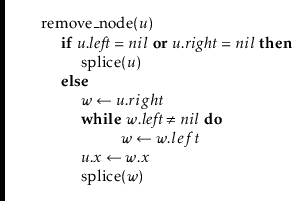Figure 6.9: Deleting a value (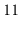) from a node,, with two children is done by replacing's value with the smallest value in the right subtree of.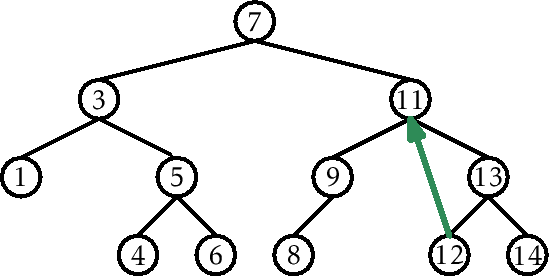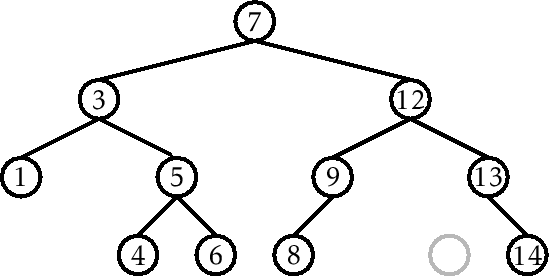## 6.2.4 Summary

The,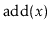, and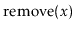operations in a BinarySearchTree each involve following a path from the root of the tree to some node in the tree. Without knowing more about the shape of the tree it is difficult to say much about the length of this path, except that it is less than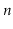, the number of nodes in the tree. The following (unimpressive) theorem summarizes the performance of the BinarySearchTree data structure:

Theorem 6..1   BinarySearchTree implements the SSet interface and supports the operations,, andin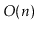time per operation.

Theorem 6.1 compares poorly with Theorem 4.1, which shows that the SkiplistSSet structure can implement the SSet interface with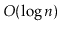expected time per operation. The problem with the BinarySearchTree structure is that it can become unbalanced. Instead of looking like the tree in Figure 6.5 it can look like a long chain ofnodes, all but the last having exactly one child.

There are a number of ways of avoiding unbalanced binary search trees, all of which lead to data structures that havetime operations. In Chapter 7 we show howexpected time operations can be achieved with randomization. In Chapter 8 we show howamortized time operations can be achieved with partial rebuilding operations. In Chapter 9 we show howworst-case time operations can be achieved by simulating a tree that is not binary: one in which nodes can have up to four children.

opendatastructures.org